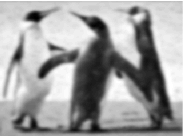GeeksforGeeks App
Open AppBrowser
Continue

# MATLAB – Butterworth Lowpass Filter in Image Processing

In the field of Image Processing, Butterworth Lowpass Filter (BLPF) is used for image smoothing in the frequency domain. It removes high-frequency noise from a digital image and preserves low-frequency components. The transfer function of BLPF of orderis defined as-Where,

•is a positive constant. BLPF passes all the frequencies less thanvalue without attenuation and cuts off all the frequencies greater than it.
• Thisis the transition point between H(u, v) = 1 and H(u, v) = 0, so this is termed as cutoff frequency. But instead of making a sharp cut-off (like, Ideal Lowpass Filter (ILPF)), it introduces a smooth transition from 1 to 0 to reduce ringing artifacts.
•is the Euclidean Distance from any point (u, v) to the origin of the frequency plane, i.e,Approach:
Step 1: Input – Read an image
Step 2: Saving the size of the input image in pixels
Step 3: Get the Fourier Transform of the input_image
Step 4: Assign the orderand cut-off frequencyStep 5: Designing filter: Butterworth Low Pass Filter
Step 6: Convolution between the Fourier Transformed input image and the filtering mask
Step 7: Take Inverse Fourier Transform of the convoluted image
Step 8: Display the resultant image as output

Implementation in MATLAB:

 % MATLAB Code | Butterworth Low Pass Filter      % Reading input image : input_imageinput_image = imread('[name of input image file].[file format]');  % Saving the size of the input_image in pixels-% M : no of rows (height of the image)% N : no of columns (width of the image)[M, N] = size(input_image);  % Getting Fourier Transform of the input_image% using MATLAB library function fft2 (2D fast fourier transform)FT_img = fft2(double(input_image));  % Assign the order valuen = 2; % one can change this value accordingly  % Assign Cut-off FrequencyD0 = 20; % one can change this value accordingly  % Designing filteru = 0:(M-1);v = 0:(N-1);idx = find(u > M/2);u(idx) = u(idx) - M;idy = find(v > N/2);v(idy) = v(idy) - N;  % MATLAB library function meshgrid(v, u) returns % 2D grid which contains the coordinates of vectors % v and u. Matrix V with each row is a copy of v % and matrix U with each column is a copy of u [V, U] = meshgrid(v, u);  % Calculating Euclidean DistanceD = sqrt(U.^2 + V.^2);  % determining the filtering maskH = 1./(1 + (D./D0).^(2*n));  % Convolution between the Fourier Transformed % image and the maskG = H.*FT_img;  % Getting the resultant image by Inverse Fourier Transform % of the convoluted image using MATLAB library function  % ifft2 (2D inverse fast fourier transform)   output_image = real(ifft2(double(G)));     % Displaying Input Image and Output Image subplot(2, 1, 1), imshow(input_image), subplot(2, 1, 2), imshow(output_image, [ ]);

Input Image –Output:Note: A Butterworth filter of order 1 has no ringing artifact. Generally ringing is imperceptible in filters of order 2. But it can become a significant factor in filters of a higher order. For a specific cut-off frequency, ringing increases with an increase in the filter order.

My Personal Notes arrow_drop_up